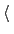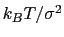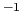Next: Statistical analysis of collective Up: Defining collective variables and Previous: Trajectory output   Contents   Index

## Extended Lagrangian.

The following options enable extended-system dynamics, where a colvar is coupled to an additional degree of freedom (fictitious particle) by a harmonic spring. All biasing and confining forces are then applied to the extended degree of freedom. The actual'' geometric colvar (function of Cartesian coordinates) only feels the force from the harmonic spring. This is particularly useful when combined with an ABF bias (13.5.1) to perform eABF simulations (13.5.2).

• extendedLagrangianAdd extended degree of freedomContext: colvar
Acceptable values: boolean
Default value: off
Description: Adds a fictitious particle to be coupled to the colvar by a harmonic spring. The fictitious mass and the force constant of the coupling potential are derived from the parameters extendedTimeConstant and extendedFluctuation, described below. Biasing forces on the colvar are applied to this fictitious particle, rather than to the atoms directly. This implements the extended Lagrangian formalism used in some metadynamics simulations .

• extendedFluctuationStandard deviation between the colvar and the fictitious particle (colvar unit)Context: colvar
Acceptable values: positive decimal
Description: Defines the spring stiffness for the extendedLagrangian mode, by setting the typical deviation between the colvar and the extended degree of freedom due to thermal fluctuation. The spring force constant is calculated internally as, whereis the value of extendedFluctuation.

• extendedTimeConstantOscillation period of the fictitious particle (fs)Context: colvar
Acceptable values: positive decimal
Default value: 200
Description: Defines the inertial mass of the fictitious particle, by setting the oscillation period of the harmonic oscillator formed by the fictitious particle and the spring. The period should be much larger than the MD time step to ensure accurate integration of the extended particle's equation of motion. The fictitious mass is calculated internally as, whereis the period andis the typical fluctuation (see above).

• extendedTempTemperature for the extended degree of freedom (K)Context: colvar
Acceptable values: positive decimal
Default value: thermostat temperature
Description: Temperature used for calculating the coupling force constant of the extended variable (see extendedFluctuation) and, if needed, as a target temperature for extended Langevin dynamics (see extendedLangevinDamping). This should normally be left at its default value.

• extendedLangevinDampingDamping factor for extended Langevin dynamics (ps)Context: colvar
Acceptable values: positive decimal
Default value: 1.0
Description: If this is non-zero, the extended degree of freedom undergoes Langevin dynamics at temperature extendedTemp. The friction force is minus extendedLangevinDamping times the velocity. This is useful because the extended dynamics coordinate may heat up in the transient non-equilibrium regime of ABF. Use moderate damping values, to limit viscous friction (potentially slowing down diffusive sampling) and stochastic noise (increasing the variance of statistical measurements). In doubt, use the default value.Next: Statistical analysis of collective Up: Defining collective variables and Previous: Trajectory output   Contents   Index
vmd@ks.uiuc.edu